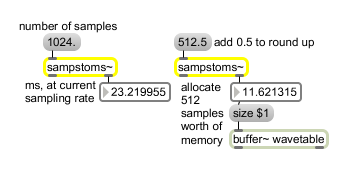sampstoms~

Convert time from samples to milliseconds

Description

sampstoms~ will convert an incoming signal carrying a time value in samples and output a signal which converts that number of samples to a number of milliseconds (at the current sampling rate).

None.

Messages

 int sample-value [int] A value representing a number of samples received in the inlet is converted to milliseconds at the current sampling rate and sent out the object's right outlet. The input may contain a fractional number of samples. For example, at 44.1 kHz sampling rate, 322.45 samples is 7.31 milliseconds. (A float or int input triggers output even when audio is off.) float sample-value [float] A value representing a number of samples received in the inlet is converted to milliseconds at the current sampling rate and sent out the object's right outlet. The input may contain a fractional number of samples. For example, at 44.1 kHz sampling rate, 322.45 samples is 7.31 milliseconds. (A float or int input triggers output even when audio is off.) signal Values in the signal represent a number of samples, and are converted to milliseconds at the current sampling rate to output as a signal out the left outlet. The input may contain a fractional number of samples.

Output

float: Out right outlet: A number of milliseconds corresponding to a number of samples received in the inlet.
signal: Out left outlet: A signal consisting of the number of milliseconds corresponding to values representing a number of samples in the input signal.

ExamplesSome objects refer to time in samples some in milliseconds